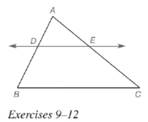Chapter 5.6, Problem 11EElementary Geometry For College St...

7th Edition
Alexander + 2 others
ISBN: 9781337614085

Solutions

Chapter
SectionElementary Geometry For College St...

7th Edition
Alexander + 2 others
ISBN: 9781337614085
Textbook Problem

Given: D E ↔ ∥ B C , ¯ A D = a − 1 , D B = 2 a + 2 , A E = a , E C = 4 a − 5 Find: a  and  A DTo determine

To find:

Explanation

Given:

Theorem used:

If a line is parallel to one side of a triangle and intersects other two sides, then it divides these sides proportionally.

Calculation:

Given: DEBC¯

Since if a line is parallel to one side of a triangle and intersects other two sides, then it divides these sides proportionally.

Also given that AD=a1,DB=2a+2,AE=a,EC=4a5.

Thus a12a+2=a4a5.

Now let us find the value of a.

a12a+2=a4a5(a1)(4a5)=a(2</

Still sussing out bartleby?

Check out a sample textbook solution.

See a sample solution

The Solution to Your Study Problems

Bartleby provides explanations to thousands of textbook problems written by our experts, many with advanced degrees!

Get Started

Find more solutions based on key concepts